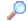Search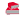Directory

Desktop Functions:

Smart Device Functions: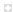Glossary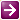Show Recent ChangesSubscribe (RSS)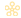Misc. Pages
GetExtendedTcpTable (iphlpapi)

Summary
The GetExtendedTcpTable function retrieves a table that contains a list of TCP endpoints available to the application.

#### C# Signature:

[DllImport("iphlpapi.dll", SetLastError=true)]
static extern uint GetExtendedTcpTable(IntPtr pTcpTable, ref int dwOutBufLen, bool sort, int ipVersion, TCP_TABLE_CLASS tblClass,uint reserved = 0);

#### VB Signature:

<DllImport("iphlpapi.dll", SetLastError:=True)> _
Public Shared Function GetExtendedTcpTable(ByVal pTcpTable As IntPtr, ByRef dwOutBufLen As Integer, ByVal sort As Boolean, ByVal ipVersion As  Integer, ByVal tblClass As TCP_TABLE_CLASS, ByVal reserved As Integer) As UInteger
End Function

TCP_TABLE_CLASS

#### Notes:

When you do not need the PID information look at IPGlobalProperties.GetActiveTcpListeners and IPGlobalProperties.GetActiveTcpConnections methods from the System.Net.NetworkInformation namespace

MS Docs:

https://docs.microsoft.com/en-us/dotnet/api/system.net.networkinformation.ipglobalproperties.getactivetcplisteners

https://docs.microsoft.com/en-us/dotnet/api/system.net.networkinformation.ipglobalproperties.getactivetcpconnections

#### Sample Code:

Generic methods for IPv4 and IPv6. Using MIB_TCPROW_OWNER_PID, MIB_TCPTABLE_OWNER_PID, MIB_TCP6ROW_OWNER_PID, MIB_TCP6TABLE_OWNER_PID and TCP_TABLE_CLASS

public const int AF_INET = 2;    // IP_v4 = System.Net.Sockets.AddressFamily.InterNetwork
public const int AF_INET6 = 23;  // IP_v6 = System.Net.Sockets.AddressFamily.InterNetworkV6

public static List<MIB_TCPROW_OWNER_PID> GetAllTCPConnections()
{
return GetTCPConnections<MIB_TCPROW_OWNER_PID, MIB_TCPTABLE_OWNER_PID>(AF_INET);
}

public static List<MIB_TCP6ROW_OWNER_PID> GetAllTCPv6Connections()
{
return GetTCPConnections<MIB_TCP6ROW_OWNER_PID, MIB_TCP6TABLE_OWNER_PID>(AF_INET6);
}

private static List<IPR> GetTCPConnections<IPR, IPT>(int ipVersion)//IPR = Row Type, IPT = Table Type
{
IPR[] tableRows;
int buffSize = 0;

var dwNumEntriesField = typeof(IPT).GetField("dwNumEntries");

// how much memory do we need?
uint ret = GetExtendedTcpTable(IntPtr.Zero, ref buffSize, true, ipVersion, TCP_TABLE_CLASS.TCP_TABLE_OWNER_PID_ALL);
IntPtr tcpTablePtr = Marshal.AllocHGlobal(buffSize);

try
{
ret = GetExtendedTcpTable(tcpTablePtr, ref buffSize, true, ipVersion, TCP_TABLE_CLASS.TCP_TABLE_OWNER_PID_ALL);
if (ret != 0)
return new List<IPR>();

// get the number of entries in the table
IPT table = (IPT)Marshal.PtrToStructure(tcpTablePtr, typeof(IPT));
int rowStructSize = Marshal.SizeOf(typeof(IPR));
uint numEntries = (uint)dwNumEntriesField.GetValue(table);

// buffer we will be returning
tableRows = new IPR[numEntries];

IntPtr rowPtr = (IntPtr)((long)tcpTablePtr + 4);
for (int i = 0; i < numEntries; i++)
{
IPR tcpRow = (IPR)Marshal.PtrToStructure(rowPtr, typeof(IPR));
tableRows[i] = tcpRow;
rowPtr = (IntPtr)((long)rowPtr + rowStructSize);   // next entry
}
}
finally
{
// Free the Memory
Marshal.FreeHGlobal(tcpTablePtr);
}
return tableRows != null ? tableRows.ToList() : new List<IPR>();
}

#### Sample Code:

Previous sample

public MIB_TCPROW_OWNER_PID[] GetAllTcpConnections()
{
MIB_TCPROW_OWNER_PID[] tTable;
int AF_INET = 2;    // IP_v4
int buffSize = 0;

// how much memory do we need?
uint ret = GetExtendedTcpTable(IntPtr.Zero, ref buffSize, true, AF_INET, TCP_TABLE_CLASS.TCP_TABLE_OWNER_PID_ALL);
IntPtr buffTable = Marshal.AllocHGlobal(buffSize);

try
{
ret = GetExtendedTcpTable(buffTable, ref buffSize, true, AF_INET, TCP_TABLE_CLASS.TCP_TABLE_OWNER_PID_ALL);
if (ret != 0)
{
return null;
}

// get the number of entries in the table
MIB_TCPTABLE_OWNER_PID tab = (MIB_TCPTABLE_OWNER_PID)Marshal.PtrToStructure(buffTable, typeof(MIB_TCPTABLE_OWNER_PID));
IntPtr rowPtr = (IntPtr)((long)buffTable + Marshal.SizeOf(tab.dwNumEntries));
tTable = new MIB_TCPROW_OWNER_PID[tab.dwNumEntries];

for (int i = 0; i < tab.dwNumEntries; i++)
{
MIB_TCPROW_OWNER_PID tcpRow = (MIB_TCPROW_OWNER_PID)Marshal.PtrToStructure(rowPtr, typeof(MIB_TCPROW_OWNER_PID));
tTable[i] = tcpRow;
rowPtr = (IntPtr)((long)rowPtr + Marshal.SizeOf(tcpRow));   // next entry
}
}
finally
{
// Free the Memory
Marshal.FreeHGlobal(buffTable);
}
return tTable;
}

#### PowerShell

\$TypeDefinition=@"
using System;
using System.Runtime.InteropServices;
using System.Collections;
using System.Collections.Generic;
using System.Linq;

// https://msdn2.microsoft.com/en-us/library/aa366073.aspx
namespace IPHelper {

// https://msdn2.microsoft.com/en-us/library/aa366913.aspx
[StructLayout(LayoutKind.Sequential)]
public struct MIB_TCPROW_OWNER_PID {
public uint state;
[MarshalAs(UnmanagedType.ByValArray, SizeConst = 4)]
public byte[] localPort;
[MarshalAs(UnmanagedType.ByValArray, SizeConst = 4)]
public byte[] remotePort;
public uint owningPid;
}

// https://msdn2.microsoft.com/en-us/library/aa366921.aspx
[StructLayout(LayoutKind.Sequential)]
public struct MIB_TCPTABLE_OWNER_PID {
public uint dwNumEntries;
[MarshalAs(UnmanagedType.ByValArray, ArraySubType = UnmanagedType.Struct, SizeConst = 1)]
public MIB_TCPROW_OWNER_PID[] table;
}

// https://msdn.microsoft.com/en-us/library/aa366896
[StructLayout(LayoutKind.Sequential)]
public struct MIB_TCP6ROW_OWNER_PID {
[MarshalAs(UnmanagedType.ByValArray, SizeConst = 16)]
public uint localScopeId;
[MarshalAs(UnmanagedType.ByValArray, SizeConst = 4)]
public byte[] localPort;
[MarshalAs(UnmanagedType.ByValArray, SizeConst = 16)]
public uint remoteScopeId;
[MarshalAs(UnmanagedType.ByValArray, SizeConst = 4)]
public byte[] remotePort;
public uint state;
public uint owningPid;
}

// https://msdn.microsoft.com/en-us/library/windows/desktop/aa366905
[StructLayout(LayoutKind.Sequential)]
public struct MIB_TCP6TABLE_OWNER_PID {
public uint dwNumEntries;
[MarshalAs(UnmanagedType.ByValArray, ArraySubType = UnmanagedType.Struct, SizeConst = 1)]
public MIB_TCP6ROW_OWNER_PID[] table;
}

// https://msdn2.microsoft.com/en-us/library/aa366386.aspx
public enum TCP_TABLE_CLASS {
TCP_TABLE_BASIC_LISTENER,
TCP_TABLE_BASIC_CONNECTIONS,
TCP_TABLE_BASIC_ALL,
TCP_TABLE_OWNER_PID_LISTENER,
TCP_TABLE_OWNER_PID_CONNECTIONS,
TCP_TABLE_OWNER_PID_ALL,
TCP_TABLE_OWNER_MODULE_LISTENER,
TCP_TABLE_OWNER_MODULE_CONNECTIONS,
TCP_TABLE_OWNER_MODULE_ALL
}

// https://msdn.microsoft.com/en-us/library/aa366896.aspx
public enum MIB_TCP_STATE {
MIB_TCP_STATE_CLOSED,
MIB_TCP_STATE_LISTEN,
MIB_TCP_STATE_SYN_SENT,
MIB_TCP_STATE_SYN_RCVD,
MIB_TCP_STATE_ESTAB,
MIB_TCP_STATE_FIN_WAIT1,
MIB_TCP_STATE_FIN_WAIT2,
MIB_TCP_STATE_CLOSE_WAIT,
MIB_TCP_STATE_CLOSING,
MIB_TCP_STATE_LAST_ACK,
MIB_TCP_STATE_TIME_WAIT,
MIB_TCP_STATE_DELETE_TCB
}

public static class IPHelperAPI {
[DllImport("iphlpapi.dll", SetLastError = true)]
internal static extern uint GetExtendedTcpTable(
IntPtr tcpTable,
ref int tcpTableLength,
bool sort,
int ipVersion,
TCP_TABLE_CLASS tcpTableType,
int reserved=0);
}

public class IPHelperWrapper : IDisposable {

public const int AF_INET = 2;    // IP_v4 = System.Net.Sockets.AddressFamily.InterNetwork
public const int AF_INET6 = 23;  // IP_v6 = System.Net.Sockets.AddressFamily.InterNetworkV6

// Creates a new wrapper for the local machine
public IPHelperWrapper() { }

// Disposes of this wrapper
public void Dispose() { GC.SuppressFinalize(this); }

public List<MIB_TCPROW_OWNER_PID> GetAllTCPv4Connections() {
return GetTCPConnections<MIB_TCPROW_OWNER_PID, MIB_TCPTABLE_OWNER_PID>(AF_INET);
}

public List<MIB_TCP6ROW_OWNER_PID> GetAllTCPv6Connections() {
return GetTCPConnections<MIB_TCP6ROW_OWNER_PID, MIB_TCP6TABLE_OWNER_PID>(AF_INET6);
}

public List<IPR> GetTCPConnections<IPR, IPT>(int ipVersion) { //IPR = Row Type, IPT = Table Type

IPR[] tableRows;
int buffSize = 0;
var dwNumEntriesField = typeof(IPT).GetField("dwNumEntries");

// how much memory do we need?
uint ret = IPHelperAPI.GetExtendedTcpTable(IntPtr.Zero, ref buffSize, true, ipVersion, TCP_TABLE_CLASS.TCP_TABLE_OWNER_PID_ALL);
IntPtr tcpTablePtr = Marshal.AllocHGlobal(buffSize);

try {
ret = IPHelperAPI.GetExtendedTcpTable(tcpTablePtr, ref buffSize, true, ipVersion, TCP_TABLE_CLASS.TCP_TABLE_OWNER_PID_ALL);
if (ret != 0) return new List<IPR>();

// get the number of entries in the table
IPT table = (IPT)Marshal.PtrToStructure(tcpTablePtr, typeof(IPT));
int rowStructSize = Marshal.SizeOf(typeof(IPR));
uint numEntries = (uint)dwNumEntriesField.GetValue(table);

// buffer we will be returning
tableRows = new IPR[numEntries];

IntPtr rowPtr = (IntPtr)((long)tcpTablePtr + 4);
for (int i = 0; i < numEntries; i++) {
IPR tcpRow = (IPR)Marshal.PtrToStructure(rowPtr, typeof(IPR));
tableRows[i] = tcpRow;
rowPtr = (IntPtr)((long)rowPtr + rowStructSize);   // next entry
}
}
finally {
// Free the Memory
Marshal.FreeHGlobal(tcpTablePtr);
}
return tableRows != null ? tableRows.ToList() : new List<IPR>();
}

// Occurs on destruction of the Wrapper
~IPHelperWrapper() { Dispose(); }

} // wrapper class
} // namespace
"@
Add-Type -TypeDefinition \$TypeDefinition -PassThru | Out-Null

function NetStat {

\$x=New-Object IPHelper.IPHelperWrapper
\$y=\$x.GetAllTCPv4Connections()
\$services=Get-WmiObject -Namespace "root\cimv2" -Class "Win32_Service"
\$output=@()
for (\$i=0; \$i -lt \$y.Count; \$i++) {
\$objOutput=New-Object -TypeName PSObject
Add-Member -InputObject \$objOutput -MemberType NoteProperty -Name "LocalPort" -Value (\$y[\$i].localPort+(\$y[\$i].localPort*0x100)+(\$y[\$i].localPort*0x1000)+(\$y[\$i].localPort*0x10000))
Add-Member -InputObject \$objOutput -MemberType NoteProperty -Name "RemotePort" -Value (\$y[\$i].remotePort+(\$y[\$i].remotePort*0x100)+(\$y[\$i].remotePort*0x1000)+(\$y[\$i].remotePort*0x10000))
Add-Member -InputObject \$objOutput -MemberType NoteProperty -Name "PID" -Value \$y[\$i].owningPid
Add-Member -InputObject \$objOutput -MemberType NoteProperty -Name "ProcessName" -Value ((Get-Process -Id \$y[\$i].owningPid).ProcessName)
Add-Member -InputObject \$objOutput -MemberType NoteProperty -Name "StateValue" -Value \$y[\$i].state
Add-Member -InputObject \$objOutput -MemberType NoteProperty -Name "State" -Value \$StateList[\$y[\$i].state]
\$boolNoService=\$true
for (\$j=0; \$j -lt \$services.Count; \$j++) {
if (\$services[\$j].ProcessId -eq \$y[\$i].owningPid) {
Add-Member -InputObject \$objOutput -MemberType NoteProperty -Name "ServiceName" -Value \$services[\$j].Caption
\$boolNoService=\$false
break;
}
}
if (\$boolNoService) { Add-Member -InputObject \$objOutput -MemberType NoteProperty -Name "ServiceName" -Value \$null }
\$output+=\$objOutput
}
\$output
}

NetStat

#### VB.NET

' supporting structures and enumerations

Public Const AF_INET As Integer = 2 'IPv4

Public Enum TCP_TABLE_CLASS
TCP_TABLE_BASIC_LISTENER = 0
TCP_TABLE_BASIC_CONNECTIONS = 1
TCP_TABLE_BASIC_ALL = 2
TCP_TABLE_OWNER_PID_LISTENER = 3
TCP_TABLE_OWNER_PID_CONNECTIONS = 4
TCP_TABLE_OWNER_PID_ALL = 5
TCP_TABLE_OWNER_MODULE_LISTENER = 6
TCP_TABLE_OWNER_MODULE_CONNECTIONS = 7
TCP_TABLE_OWNER_MODULE_ALL = 8
End Enum

<StructLayout(LayoutKind.Sequential)> _
Public s_b1 As Byte
Public s_b2 As Byte
Public s_b3 As Byte
Public s_b4 As Byte
End Structure

<StructLayout(LayoutKind.Explicit)> _
Public Structure MIB_TCPTABLE_OWNER_PID
Public dwNumEntries As UInteger
Public table() As MIB_TCPROW_OWNER_PID
End Structure

' note: functions added to this struct to demonstrate how to convert the addresses and ports into the form you would want them

<StructLayout(LayoutKind.Explicit)> _
Public Structure MIB_TCPROW_OWNER_PID
Public dwState As UInteger
Public dwLocalPort As UInteger
Public dwRemotePort As UInteger
Public dwOwningPid As UInteger
End Function
Public Function LocalPort() As Integer
If dwLocalPort > 0 Then
Dim b() As Byte = BitConverter.GetBytes(dwLocalPort)
Array.Reverse(b, 0, 2)
Return BitConverter.ToInt32(b, 0)
Else
Return 0
End If
End Function
End Function
Public Function RemotePort() As Integer
If dwRemotePort > 0 Then
Dim b() As Byte = BitConverter.GetBytes(dwRemotePort)
Array.Reverse(b, 0, 2)
Return BitConverter.ToInt32(b, 0)
Else
Return 0
End If
End Function
End Structure

' This example gets a list of all TCP connections and their owner PIDs

' Credit: partially based upon the C# example above

Public Sub GetExtendedTcpTableExample()

Dim dwNumEntriesField As System.Reflection.FieldInfo = GetType(MIB_TCPTABLE_OWNER_PID).GetField("dwNumEntries")
Dim BufLen As Integer = 0
' Because we have to marshal our structure pointer to a specific size, we'll have to call the function twice.
' The first time dumps the output into a null pointer, but it lets us get the buffer size we need to create the real pointer.
Dim Ret As UInteger = GetExtendedTcpTable(IntPtr.Zero, BufLen, True, AF_INET, TCP_TABLE_CLASS.TCP_TABLE_OWNER_PID_ALL, 0)
Dim TblPtr As IntPtr = Marshal.AllocHGlobal(BufLen)

' Call it again with our structure pointer and known buffer size in place
Ret = GetExtendedTcpTable(TblPtr, BufLen, True, AF_INET, TCP_TABLE_CLASS.TCP_TABLE_OWNER_PID_ALL, 0)

' convert the pointer into a table structure
Dim TblStruct As MIB_TCPTABLE_OWNER_PID = Marshal.PtrToStructure(TblPtr, GetType(MIB_TCPTABLE_OWNER_PID))
Dim RowStructSize As Integer = Marshal.SizeOf(GetType(MIB_TCPROW_OWNER_PID))
Dim TcpTableRows As New List(Of MIB_TCPROW_OWNER_PID)
' the pointer for the array holding the table rows is set to the end of the table pointer + the memory alignment offset of the row struct (if any)
Dim RowArrayPtr As IntPtr = CType((CLng(TblPtr) + CLng(Marshal.OffsetOf(GetType(MIB_TCPTABLE_OWNER_PID), "table"))), IntPtr)

For i As Integer = 0 To TblStruct.dwNumEntries
Dim TableRow As MIB_TCPROW_OWNER_PID = Marshal.PtrToStructure(RowArrayPtr, GetType(MIB_TCPROW_OWNER_PID))

", LocalPort:" & TcpTableRows.Last.LocalPort.ToString & _
", RemotePort:" & TcpTableRows.Last.RemotePort.ToString & _
", PID:" & TcpTableRows.Last.dwOwningPid)

RowArrayPtr = CType((CLng(RowArrayPtr) + RowStructSize), IntPtr)
Next

End Sub

Documentation

Do you have...

• helpful tips or sample code to share for using this API in managed code?
• corrections to the existing content?
• variations of the signature you want to share?
• additional languages you want to include?

Select "Edit This Page" on the right hand toolbar and edit it! Or add new pages containing supporting types needed for this API (structures, delegates, and more).Access PInvoke.net directly from VS:Terms of UseEdit This PageFind ReferencesShow Printable Version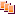Revisions Show changes